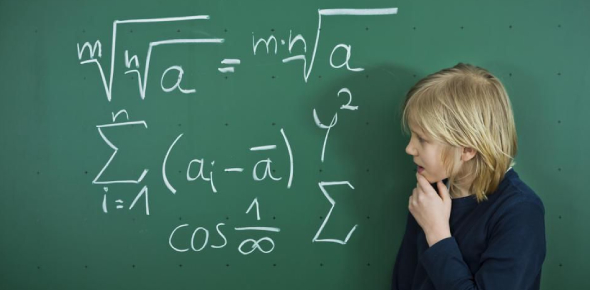# SAT: Math Practice Quiz! Test

16 Questions | Attempts: 621Settings.

• 1.
If (1/3)y + 9 = 0, then y =
• A.

-27

• B.

-9

• C.

-3

• D.

3

• E.

27

• 2.
In the figure above, P, Q, and R lie on the same line. P is the center of the larger circle, and Q is the center of the smaller circle. If the radius of the larger circle is 4, what is the radius of the smaller circle?
• A.

1

• B.

2

• C.

4

• D.

8

• E.

16

• 3.
Roy planted corn on 1/5 of his land. If he planted 45 acres of corn, how many acres of land does he have?
• A.

90

• B.

112 1/2

• C.

135

• D.

225

• E.

337 1/2

• 4.
6, 10, 18, 34, 66 The first number in the list above is 6. Which of the following gives a rule for finding each successive number in the list?
• A.

Add 4 to the preceding number.

• B.

Take 1/2 of the preceding number and then add 7 to that result.

• C.

Double the preceding number and then subtract 2 from that result.

• D.

Subtract 2 from the preceding number and then double that result.

• E.

Triple the preceding number and then subtract 8 from that result.

• 5.
The two semicircles in the figure above have centers R and S, respectively. If RS = 12, what is the total length of the darkened curve?
• A.

• B.

• C.

12π

• D.

15π

• E.

16π

• 6.
If h and k are positive numbers and h + k = 7, then (7 - k)/h =
• A.

1

• B.

0

• C.

-1

• D.

H

• E.

K - 1

• 7.
The table above shows the populations of two countries and their population densities. The number of square miles in the area of Country B is approximately how much greater than the number of square miles in the area of Country A ?
• A.

200

• B.

3,600

• C.

5,000

• D.

8,000

• E.

950,000,000

• 8.
If x2 = x + 6, which of the following must be true?
• A.

X = 6

• B.

X< 3

• C.

X > 0

• D.

X^2 < x

• E.

X^2 > x

• 9.
Let the functions f be defined by f(x) = 5x - 2a, where a is a constant. If f(10) + f(5) = 55, what is the value of a?
• A.

-5

• B.

0

• C.

5

• D.

10

• E.

20

• 10.
A number is called “even-odd” if it is halfway between an even integer and an odd integer. If x is an even-odd number, which of the following must be true? I. 2x is an integer. II. 2x is even-odd. III. x is halfway between two even integers.
• A.

I only

• B.

II only

• C.

I and II only

• D.

II and III only

• E.

I, II, and III

• 11.
If m is a positive integer, which of the following is NOT equal to (24)m ?
• A.

2^(4m)

• B.

4^(2m)

• C.

2^m(2^(3m))

• D.

4^m(2^m)

• E.

16^m

• 12.
In the figure above, l || m. Which of the following must equal 180?
• A.

K + n + r

• B.

K + p + s

• C.

N + p + s

• D.

P + n + t

• E.

R + s + t

• 13.
How many different ordered pairs (x, y) are there such that x is an even integer, where 4 ≤ x ≤ 10, and y is an integer, where 4 < y < 10 ?
• A.

8

• B.

12

• C.

20

• D.

30

• E.

36

• 14.
N(t) = 500(0.81)t The function above can be used to model the population of a certain endangered species of animal. If n(t) gives the number of the species living t decades after the year 1900, which of the following is true about the population of the species from 1900 to 1920 ?
• A.

• B.

• C.

• D.

• E.

• 15.
A sphere of radius r inside a cube touches each one of the six sides of the cube. What is the volume of the cube, in terms of r ?
• A.

R^3

• B.

2r^3

• C.

4r^3

• D.

(4/3)r^3

• E.

8r^3

• 16.
According to the graph above, in which year was the ratio of the number of students enrolled at School B  to the number of students enrolled at School A the greatest?
• A.

1990

• B.

1991

• C.

1992

• D.

1993

• E.

1994

## Related TopicsBack to top
×

Wait!
Here's an interesting quiz for you.